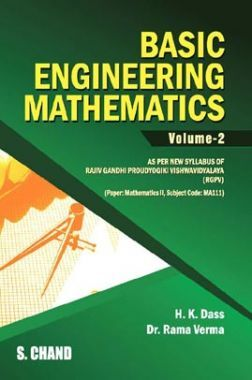•19% Off

# Basic Engineering Mathematics Volume - II (For 3rd Semester of RGPV, Bhopal)

By H. K. Dass, Verma Rama more
44 Views
Selling Price : ₹226.80
MRP : ₹280.00
You will save : ₹53.20 after 19% Discount

Save extra with 3 Offers

Get ₹ 50

Instant Cashback on the purchase of ₹ 400 or above

NEW35

Get Flat 35% Off on your First Order

Product Specifications

 Publisher SChand Publications All Engineering Mathematics books by SChand Publications ISBN 9789352531363 Author: H. K. Dass, Verma Rama Number of Pages 377 Edition Seventh Revised Edition Available Available in all digital devicesBasic Engineering Mathematics Volume - II (For 3rd Semester of RGPV, Bhopal) by H. K. Dass, Verma Rama Book Summary: This book is revised according to the new syllabus of mathematics of second semester for all Engineering colleges affiliated to Rajiv Gandhi Proudyogiki Vishwavidyalaya, Bhopal (M.P.). This book also covers the B. Tech., (B.E.) B. Arch. II semester course of other Indian Engineering colleges. This book is divided into 12 chapters on the topics of the syllabus so that the students can easily understand the subject. Audience of the Book : This book Useful for Engineering Students. Table of Content: 1. Rank Of Matrix 2. Solution Of Simultaneous Equation 3. Eigen Values, Eigen Vector, Cayley Hamilton Theorem 4. First Order Differential Equations 5. Linear Differential Equation Which Constant Co-efficients 6. Homogeneous Linear Differential-equations 7. Simultaneous Linear Differential-equations 8. Second Order Linear Differential Equations With Variable Co-efficients 9. Linear Partial Differential Equation Of First Order 10. Non-linear Partial Differential Equation Of First Order 11. Partial Differential Equations With Constant Coefficients 12. The Method Of Separable Variables 12. Working Capital Management—Inventory Management 13. Working Capital Management—Cash Management 14. Sources of Finance 15. Ratio Analysis 16. Cash Flow Statement 17. Funds Flow Statement

Related Engineering Mathematics Books
x